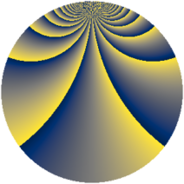# Properties

 Label 1148.2.bpLevel $1148$ Weight $2$ Character orbit 1148.bp Rep. character $\chi_{1148}(79,\cdot)$ Character field $\Q(\zeta_{24})$ Dimension $1312$ Sturm bound $336$

# Learn more about

## Defining parameters

 Level: $$N$$ $$=$$ $$1148 = 2^{2} \cdot 7 \cdot 41$$ Weight: $$k$$ $$=$$ $$2$$ Character orbit: $$[\chi]$$ $$=$$ 1148.bp (of order $$24$$ and degree $$8$$) Character conductor: $$\operatorname{cond}(\chi)$$ $$=$$ $$1148$$ Character field: $$\Q(\zeta_{24})$$ Sturm bound: $$336$$

## Dimensions

The following table gives the dimensions of various subspaces of $$M_{2}(1148, [\chi])$$.

Total New Old
Modular forms 1376 1376 0
Cusp forms 1312 1312 0
Eisenstein series 64 64 0

## Trace form

 $$1312q - 4q^{2} - 8q^{5} - 16q^{6} - 16q^{8} - 8q^{9} + O(q^{10})$$ $$1312q - 4q^{2} - 8q^{5} - 16q^{6} - 16q^{8} - 8q^{9} - 8q^{10} + 20q^{12} - 32q^{13} + 4q^{14} - 8q^{16} - 8q^{17} - 8q^{18} - 16q^{21} - 64q^{22} - 28q^{24} - 4q^{26} + 8q^{28} - 32q^{29} - 28q^{30} + 16q^{32} - 8q^{33} - 16q^{34} - 48q^{36} - 16q^{37} - 16q^{38} - 32q^{41} - 16q^{42} + 28q^{44} + 52q^{46} + 120q^{48} - 48q^{49} + 8q^{50} - 92q^{52} - 8q^{53} - 84q^{54} + 8q^{56} - 64q^{57} - 32q^{58} + 20q^{60} - 8q^{61} + 8q^{65} + 28q^{68} + 16q^{69} - 244q^{70} - 8q^{73} - 32q^{74} - 296q^{76} - 16q^{77} - 240q^{78} + 24q^{80} + 44q^{82} - 80q^{84} - 96q^{85} + 40q^{88} - 8q^{89} - 40q^{90} + 144q^{92} + 40q^{93} - 136q^{94} - 20q^{96} - 32q^{97} - 168q^{98} + O(q^{100})$$

## Decomposition of $$S_{2}^{\mathrm{new}}(1148, [\chi])$$ into newform subspaces

The newforms in this space have not yet been added to the LMFDB.